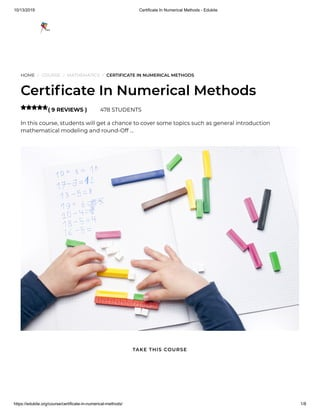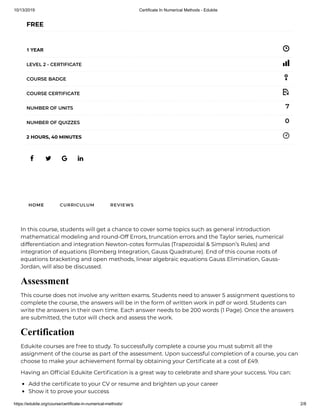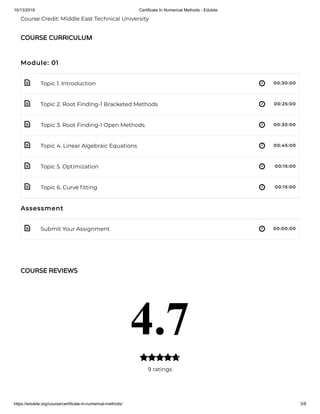Diese Präsentation wurde erfolgreich gemeldet.

# Certificate In Numerical Methods - Edukite×

1 von 8
1 von 8

# Certificate In Numerical Methods - Edukite

In this course, students will get a chance to cover some topics such as general introduction mathematical modeling and round-Off Errors, truncation errors and the Taylor series, numerical differentiation and integration Newton-cotes formulas (Trapezoidal & Simpson’s Rules) and integration of equations (Romberg Integration, Gauss Quadrature).
See More: https://bit.ly/2B9YeVX

In this course, students will get a chance to cover some topics such as general introduction mathematical modeling and round-Off Errors, truncation errors and the Taylor series, numerical differentiation and integration Newton-cotes formulas (Trapezoidal & Simpson’s Rules) and integration of equations (Romberg Integration, Gauss Quadrature).
See More: https://bit.ly/2B9YeVX

### Certificate In Numerical Methods - Edukite

1. 1. 10/13/2019 Certificate In Numerical Methods - Edukite https://edukite.org/course/certificate-in-numerical-methods/ 1/8 HOME / COURSE / MATHEMATICS / CERTIFICATE IN NUMERICAL METHODS Certi cate In Numerical Methods ( 9 REVIEWS ) 478 STUDENTS In this course, students will get a chance to cover some topics such as general introduction mathematical modeling and round-Off …  TAKE THIS COURSE